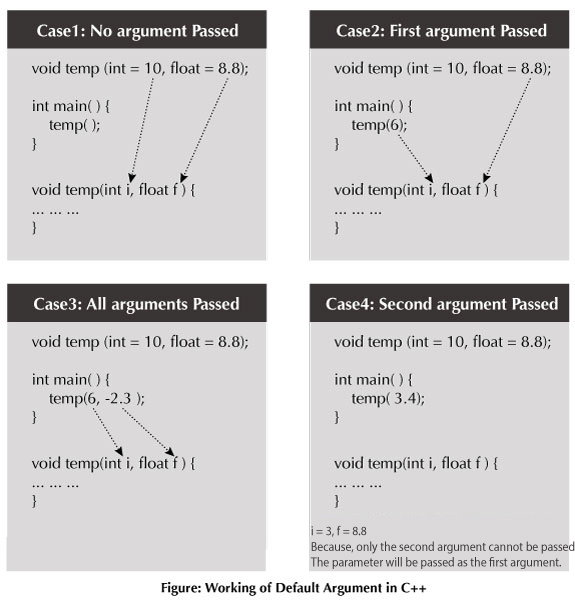C++ Programming Default Arguments (Parameters)

# C++ Programming Default Arguments (Parameters)

#### In this article, you'll learn what are default arguments, how are they used and necessary declaration for its use.

In C++ programming, you can provide default values for function parameters.

The idea behind default argument is simple. If a function is called by passing argument/s, those arguments are used by the function.

But if the argument/s are not passed while invoking a function then, the default values are used.

Default value/s are passed to argument/s in the function prototype.

## Working of default arguments## Example: Default Argument

``````// C++ Program to demonstrate working of default argument

#include <iostream>
using namespace std;

void display(char = '*', int = 1);

int main()
{
cout << "No argument passed:\n";
display();

cout << "\nFirst argument passed:\n";
display('#');

cout << "\nBoth argument passed:\n";
display('\$', 5);

return 0;
}

void display(char c, int n)
{
for(int i = 1; i <= n; ++i)
{
cout << c;
}
cout << endl;
}
``````

Output

```No argument passed:
*

First argument passed:
#

Both argument passed:
\$\$\$\$\$
```

In the above program, you can see the default value assigned to the arguments `void display(char = '*', int = 1);`.

At first, `display()` function is called without passing any arguments. In this case, `display()` function used both default arguments `c = *` and `n = 1`.

Then, only the first argument is passed using the function second time. In this case, function does not use first default value passed. It uses the actual parameter passed as the first argument `c = #` and takes default value `n = 1` as its second argument.

When `display()` is invoked for the third time passing both arguments, default arguments are not used. So, the value of `c = \$` and `n = 5.`

## Common mistakes when using Default argument

1. `void add(int a, int b = 3, int c, int d = 4);`

The above function will not compile. You cannot miss a default argument in between two arguments.
In this case, c should also be assigned a default value.

2. `void add(int a, int b = 3, int c, int d);`

The above function will not compile as well. You must provide default values for each argument after b.
In this case, c and d should also be assigned default values.
If you want a single default argument, make sure the argument is the last one. `void add(int a, int b, int c, int d = 4);`

3. No matter how you use default arguments, a function should always be written so that it serves only one purpose.
If your function does more than one thing or the logic seems too complicated, you can use Function overloading to separate the logic better.